Want to share your content on R-bloggers? click here if you have a blog, or here if you don't.

Florianne Verkroost is a Ph.D. candidate at Nuffield College at the University of Oxford. She has a passion for data science and a background in mathematics and econometrics. She applies her interdisciplinary knowledge to computationally address societal problems of inequality.

This is the third post in a series devoted to comparing different machine learning methods for predicting clothing categories from images using the Fashion MNIST data by Zalando. In the first post of this series, we prepared the data for analysis and used my “go-to” Python deep learning neural network model to predict the clothing categories of the Fashion MNIST data. In Part 2, we used principal components analysis (PCA) to compress the clothing image data down from 784 to just 17 pixels. In this post, we pick up where we left off in Part 2 and use the two data sets `train.data.pca` and `test.data.pca` to build and compare random forest and gradient-boosted models. The R code for this post can be found on my Github repository.

### Tree-Based Methods

Tree-based methods stratify or segment the predictor space into a number of simple regions using a set of decision rules that can be summarized in a decision tree. The focus here will be on classification trees, as the Fashion MNIST outcome variable is categorical with ten classes. Because single trees have a relatively low level of predictive accuracy compared to other classification approaches, I will not show you how to fit a single tree in this blog post, but you can find the code for this (as well as tree pruning) on my Github.

Ensemble methods improve predictive accuracy and decrease variance by aggregating many single decision trees. Here, I show both random forests and gradient-boosted trees as ensemble methods because the former are easier to implement as they are more robust to overfitting and require less tuning, while the latter generally outperform other tree-based methods in terms of prediction accuracy. The models are estimated in supervised mode here as labeled data are available and the goal is to predict classes. For a more formal explanation of the tree-based methods, I refer you to James et al. (2013).

### Random Forest

Random forests use bootstrap aggregating to reduce the variance of the outcomes. In the first step, bootstrapping (sampling with replacement) is used to create `B` training sets from the population with the same size as the original training set. Hereafter, a separate tree for each of these training sets is built. Trees are grown using recursive binary splitting on the training data until a node reaches some minimum number of observations. The idea is that the tree should go from impure (equal mixing of classes) to pure (each leaf corresponds to one class exactly). The splits are determined such that they decrease variance, error and impurity. Random forests decorrelate the trees by considering only `m` of all `p` predictors as split candidates, whereby often `m = sqrt(p)`.

Classification trees predict that each observation belongs to the most commonly occurring class (i.e. majority vote) of training observations in the region to which it belongs. The classification error rate is the fraction of the number of misclassified observations and the total number of classified observations. The Gini index and cross-entropy measures determine the level of impurity in order to decide on the best split at each node. In the final step, the average of the classification prediction results of all `B` trees is computed from the majority vote. The accuracy is computed as the out-of-bag (OOB) error and/or the test set error.

As each bootstrap samples from the training set with replacement, about 23 of the observations are not sampled and some are sampled multiple times. In the case of `B` trees in the forest, each observation is left out of approximately `B`/ 3 trees. The non-sampled observations are used as test set and the `B`/ 3 trees are used for out-of-sample predictions. In random forests, pruning is not needed as potential over-fitting is (partially) mitigated by the usage of bootstrapped samples and multiple decorrelated random trees.

We start by tuning the number of variables that are randomly sampled as candidates at each split,`mtry`. We make use of the `caret` framework, which makes it easy to train and evaluate a large number of different types of models. For random forests, we have the `repeatedcv` method perform five-fold cross-validation with five repetitions. For now, we build a random forest containing 200 trees because previous analyses with these data showed that the error does not decrease substantially when the number of trees is larger than 200, while a larger number of trees does require more computational power. We will see later on that 200 trees is indeed sufficient for this analysis. We let the algorithm determine what the best model is based on the accuracy metric, and we ask the algorithm to run the model for `pca.dims` (= 17) different values of `mtry`. We first specify the controls in `rf_rand_control`: we perform 5-fold cross-validation with 5 repeats (`method = "cv"`, `number = 5` and `repeats = 5`), allow parallel computation (`allowParallel = TRUE`) and save the predicted values (`savePredictions = TRUE`).

```library(caret)
rf_rand_control = trainControl(method = "repeatedcv",
search = "random",
number = 5,
repeats = 5,
allowParallel = TRUE,
savePredictions = TRUE)
set.seed(1234)
rf_rand = train(x = train.images.pca,
y = train.data.pca\$label,
method = "rf",
ntree = 200,
metric = "Accuracy",
trControl = rf_rand_control,
tuneLength = pca.dims)

print(rf_rand)
```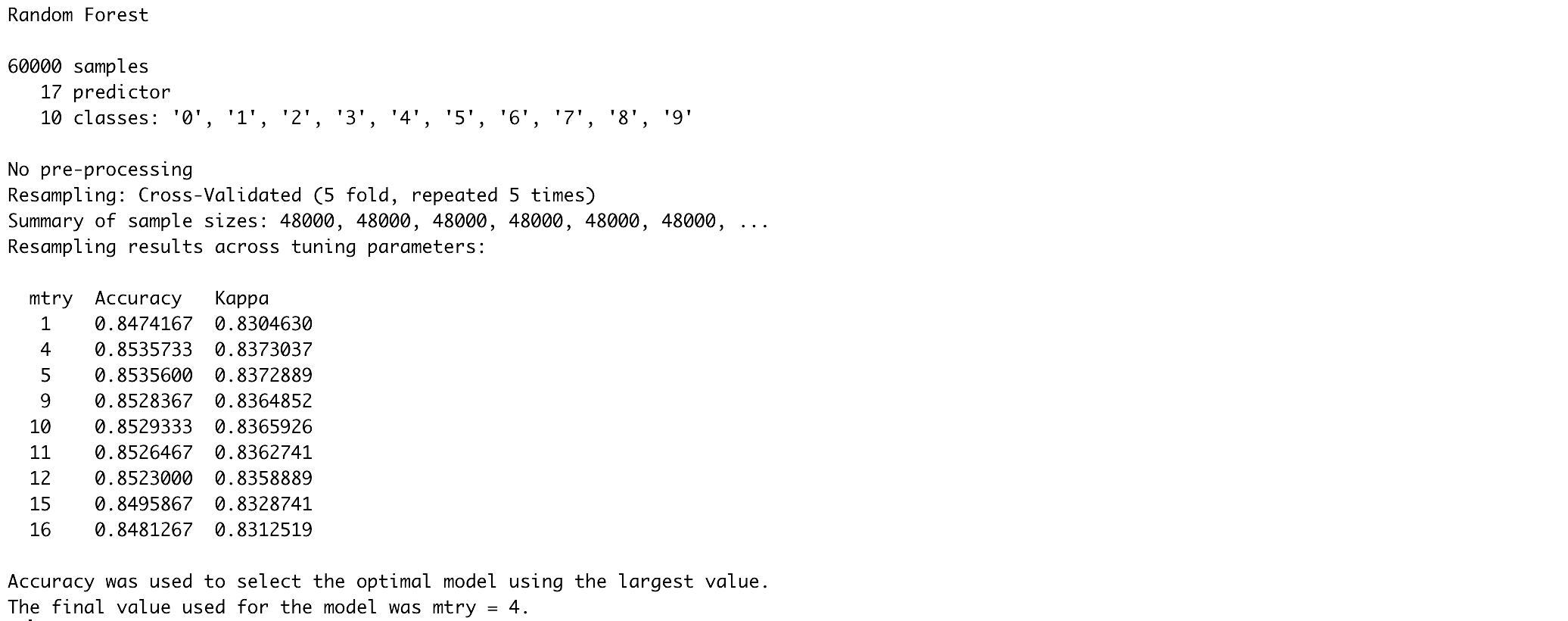We can check the model performance on both the training and test sets by means of different metrics using a custom function, `model_performance`, which can be found on my Github.

```mp.rf.rand = model_performance(rf_rand, train.images.pca, test.images.pca,
train.data.pca\$label, test.data.pca\$label, "random_forest_random")
```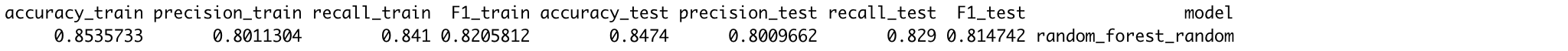We can also use the `caret` framework to perform a grid search with pre-specified values for `mtry` rather than a random search as above.

```rf_grid_control = trainControl(method = "repeatedcv",
search = "grid",
number = 5,
repeats = 5,
allowParallel = TRUE,
savePredictions = TRUE)
set.seed(1234)
rf_grid = train(x = train.images.pca,
y = train.data.pca\$label,
method = "rf",
ntree = 200,
metric = "Accuracy",
trControl = rf_grid_control,
tuneGrid = expand.grid(.mtry = c(1:pca.dims)))

plot(rf_grid)
``````mp.rf.grid = model_performance(rf_grid, train.images.pca, test.images.pca,
train.data.pca\$label, test.data.pca\$label, "random_forest_grid")
```As shown by the results, the random search selects `mtry=4` as the optimal parameter, resulting in 85% training and test set accuracies. The grid search selects `mtry=5` and achieves similar accuracies for both values of 4 and 5 for `mtry`. We can see from the results that according to `rf_rand`, `mtry` values of 4 and 5 lead to very similar results, which also goes for `mtry` values of 5 and 6 for `rf_grid`. Although the results of `rf_rand` and `rf_grid` are very similar, we choose the best model on the basis of accuracy and save this in `rf_best`. For this model, we’ll look at the relationship between the error and random forest size as well as the receiver operating characteristic (ROC) curves for every class. Let’s start by subtracting the best performing model from `rf_rand` and `rf_grid`.

```rf_models = list(rf_rand\$finalModel, rf_grid\$finalModel)
rf_accs = unlist(lapply(rf_models, function(x){ sum(diag(x\$confusion)) / sum(x\$confusion) }))
rf_best = rf_models[[which.max(rf_accs)]]
```

Next, we plot the relationship between the size of the random forest and the error using the `plot()` function from the `randomForest` package.

```library(randomForest)
plot(rf_best, main = "Relation between error and random forest size")
```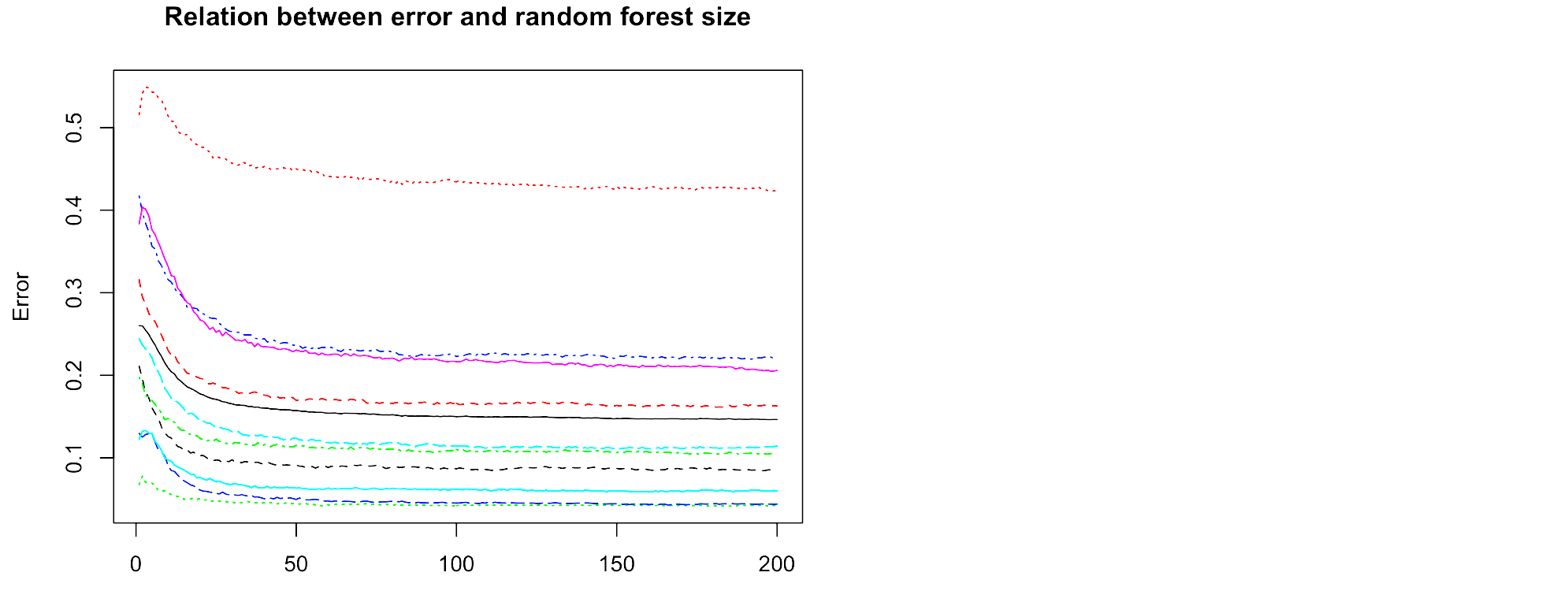We observe from this plot that the error does not decrease anymore for any of the classes after about 100 trees, and so we can conclude that our forest size of 200 is sufficient. We can also use the `varImpPlot()` function from the `randomForest` package to plot the importance for each variable. I will not show that here because it’s not as meaningful given that our variables are principal components of the actual pixels, but it’s good to keep in mind when extending these analyses to other data.

Finally, we plot the ROC curves for every class. On the x-axis of an ROC plot, we usually have the false positive rate (false positive / (true negative + false positive)) and on the y-axis the true positive rate (true positive / (true positive + false negative)). Essentially, the ROC plot helps us to compare the performance of our model with respect to predicting different classes. The area underneath each curve is the proportion of correct classifications for that particular class. Therefore, the further the curve is “drawn” towards the top left from the 45 degrees line, the better the classification for that class. We first need to obtain the data for the ROC curve for every class (or clothing category) in our data, which we bind together by rows, including a label for the classes.

```library(ROCR)
library(plyr)
pred_roc = predict(rf_best, test.images.pca, type = "prob")
classes = unique(test.data.pca\$label)
classes = classes[order(classes)]
plot_list = list()
for (i in 1:length(classes)) {
actual = ifelse(test.data.pca\$label == classes[i], 1, 0)
pred = prediction(pred_roc[, i], actual)
perf = performance(pred, "tpr", "fpr")
plot_list[[i]] = data.frame(matrix(NA, nrow = length([email protected][]), ncol = 2))
plot_list[[i]]['x'] = [email protected][]
plot_list[[i]]['y'] = [email protected][]
}
plotdf = rbind.fill(plot_list)
plotdf["Class"] = rep(cloth_cats, unlist(lapply(plot_list, nrow)))
```

Next, we plot the ROC curves for every class. Note that we use the custom plotting theme `my_theme()` as defined in the the second blog post of this series.

```ggplot() +
geom_line(data = plotdf, aes(x = x, y = y, color = Class)) +
labs(x = "False positive rate", y = "True negative rate", color = "Class") +
ggtitle("ROC curve per class") +
theme(legend.position = c(0.85, 0.35)) +
coord_fixed() +
my_theme()
```We observe from the ROC curves that shirts and pullovers are most often misclassified, whereas trousers, bags, boots and sneakers are most often correctly classified. A possible explanation for this could be that shirts and pullovers can be very similar in shape to other categories, such as tops, coats and dresses; whereas bags, trousers, boots and sneakers are more dissimilar to other categories in the data.

While in random forests each tree is fully grown and trained independently with a random sample of data, in boosting every newly built tree incorporates the error from the previously built tree. That is, the trees are grown sequentially on an adapted version of the initial data, which does not require bootstrap sampling. Because of this, boosted trees are usually smaller and more shallow than the trees in random forests, improving the tree where it does not work well enough yet. Boosting is often said to outperform random forests, which is mainly because the approach learns slowly. This learning rate can be controlled by the shrinkage parameter, which we’ll tune later.

In boosting, it’s important to tune the parameters well and play around with different values of the parameters, which can easily be done using the `caret` framework. These parameters include the learning rate, `eta`, the minimal required loss reduction to further partition on a leaf node of the tree, `gamma`, the maximal depth of a tree `max_depth`, the number of trees in the forest, `nrounds`, the minimum number of observations in the trees’ nodes, `min_child_weight`, the fraction of the training set observations randomly selected to grow trees, `subsample`, and the proportion of independent variables to use for each tree, `colsample_bytree`. An overview of all parameters can be found here. Again, we use the `caret` framework to tune our boosting model.

```xgb_control = trainControl(
method = "cv",
number = 5,
classProbs = TRUE,
allowParallel = TRUE,
savePredictions = TRUE
)
```

Next, we define the possible combinations of the tuning parameters in the form of a grid, named `xgb_grid`.

```xgb_grid = expand.grid(
nrounds = c(50, 100),
max_depth = seq(5, 15, 5),
eta = c(0.002, 0.02, 0.2),
gamma = c(0.1, 0.5, 1.0),
colsample_bytree = 1,
min_child_weight = c(1, 2, 3),
subsample = c(0.5, 0.75, 1)
)
```

We set the seed and then train the model onto the transformed principal components of the training data using `xgb_control` and `xgb_grid` as specified earlier. Note that because of the relatively large number of tuning parameters, and thus the larger number of possible combinations of these parameters (`nrow(xgb_grid) = 486`), this may take quite a long time to run.

```set.seed(1234)
xgb_tune = train(x = train.images.pca,
y = train.classes,
method = "xgbTree",
trControl = xgb_control,
tuneGrid = xgb_grid
)
xgb_tune
```

(Note that the output of xgb_tune has been truncated for this post.)Let’s have a look at the tuning parameters resulting in the highest accuracy, and the model performance overall.

```xgb_tune\$results[which.max(xgb_tune\$results\$Accuracy), ]
``````mp.xgb = model_performance(xgb_tune, train.images.pca, test.images.pca,
train.classes, test.classes, "xgboost")
```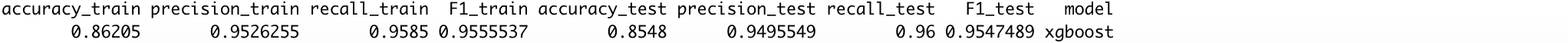The optimal combination of tuning parameter values resulted in 86.2% training and 85.5% testing accuracies. Although there may be some slight overfitting going on, the model performs a bit better than the random forest, as was expected. Let’s have a look at the confusion matrix for the test set predictions to observe what clothing categories are mostly correctly or wrongly classified.

```table(pred = predict(xgb_tune, test.images.pca),
true = test.classes)
```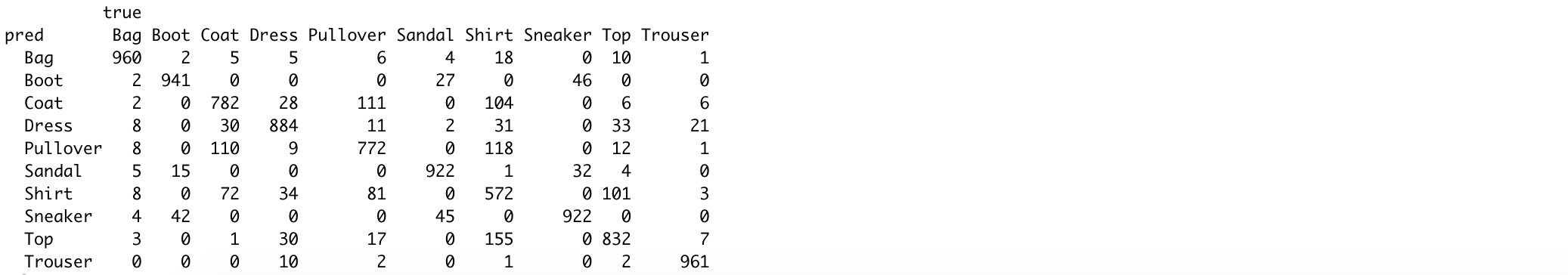As we saw with the random forests, pullovers, shirts and coats are most often mixed up, while trousers, boots, bags and sneakers are most often correctly classified.

In the next and final post of this series, we will use the PCA reduced data again, but this time to estimate and assess support vector machines. Will these models be able to achieve similar results on the reduced data as neural networks on the full data? Let’s see!